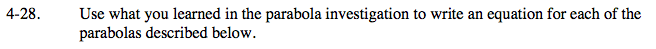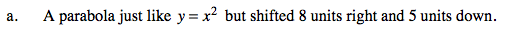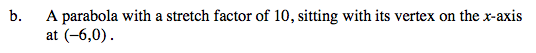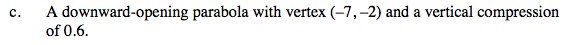### Home > A2C > Chapter Ch4 > Lesson 4.1.2 > Problem4-28

4-28.
1. Use what you learned in the parabola investigation to write an equation for each of the parabolas described below. 4-28 HW eTool (Desmos). Homework Help ✎

1. A parabola just like y = x2 but shifted 8 units right and 5 units down.

2. A parabola with a stretch factor of 10, sitting with its vertex on the x-axis at (−6, 0).

3. A downward-opening parabola with vertex (−7, −2) and a vertical compression of 0.6.y = (x − 8)2 − 5y = 10(x + 6)2Use the same method as parts (a) and (b).

Type functions for each part in the eTool below. Scroll down in the eTool to check your answers!
Click the link at right for the full version of the eTool: A2C 4-28 HW eTool